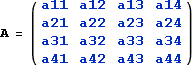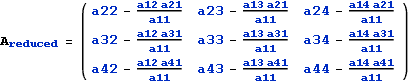# Using Node-Voltage method in the phasor domain

I was wondering if there is an easier way to solve circuits using matrix inversion if I have complex numbers. So far I've been doing them by hand with 2x2 matrices. It isn't hard, but it takes a while. I have a test coming up in about a week which will have 10 questions that have to be solved in 1 hour. My teacher said that I may have to solve 3x3 or 4x4 matrices. If I have to do it by hand I don't think I'll finish on time

## Answers and Replies

The Electrician
Gold Member
If you can have a laptop with you, your school probably has Matlab or something like it on the school network.

If you can't do that, you better get yourself a calculator that can do complex matrices, such as a TI89 or an HP48G, HP49G+ or HP50G.

You'll never be able to do a 4x4!K^2
Science Advisor
You'll never be able to do a 4x4!Gaussian elimination works just fine for 4x4 matrices. It wouldn't be fast, but it works.

Alternatively, do dimension reduction to get to a 3x3 matrix. Observe that if $\sum_{j=1}^NA_{ij}x_j=b_i$ then the following holds for x1.

$$x_1 = \frac{b_1 - \sum_{j=2}^NA_{1j}x_j}{A_{11}}$$

If you substitute this back into the system, you get the reduced system.

$$\sum_{j=2}^N\left(A_{ij}-\frac{A_{i1}A_{1j}}{A_{11}}\right)x_j=b_i-\frac{b_1A_{i1}}{A_{11}}$$

This will let you reduce a 4x4 to 3x3 and 3x3 to 2x2.

3x3 matrix can also be attacked directly. Cramer's rule for 3x3 is not that bad. It's just 1 3x3 determinant and 9 2x2 determinants. Even with complex numbers, not all that terrible. It does involve 9 complex divisions, though, to get the final result.

None of this is trivial, but it's not that complicated and can be done even with regular calculator. If you have one that does complex numbers, you can fly through them. And remember, people used to do this stuff with logarithmic slide rules. Be glad you are not them.

your school probably has Matlab or something like it
Octave does anything Matlab does and is free.

The Electrician
Gold Member
Gaussian elimination works just fine for 4x4 matrices. It wouldn't be fast, but it works.

Alternatively, do dimension reduction to get to a 3x3 matrix. Observe that if $\sum_{j=1}^NA_{ij}x_j=b_i$ then the following holds for x1.

$$x_1 = \frac{b_1 - \sum_{j=2}^NA_{1j}x_j}{A_{11}}$$

If you substitute this back into the system, you get the reduced system.

$$\sum_{j=2}^N\left(A_{ij}-\frac{A_{i1}A_{1j}}{A_{11}}\right)x_j=b_i-\frac{b_1A_{i1}}{A_{11}}$$

This will let you reduce a 4x4 to 3x3 and 3x3 to 2x2.

3x3 matrix can also be attacked directly. Cramer's rule for 3x3 is not that bad. It's just 1 3x3 determinant and 9 2x2 determinants. Even with complex numbers, not all that terrible. It does involve 9 complex divisions, though, to get the final result.

None of this is trivial, but it's not that complicated and can be done even with regular calculator. If you have one that does complex numbers, you can fly through them. And remember, people used to do this stuff with logarithmic slide rules. Be glad you are not them.

I think you're being overly optimistic. He said he does the computations "by hand" (so far), and he says that even a 2x2 "takes a while". He will have to do 10 such problems, some of which involve 3x3 and 4x4 complex matrices, in an hour. If he does them "by hand", as he has done so far, carefully so as to make no numerical mistakes, I think he's unlikely to get them all done in an hour.

Octave does anything Matlab does and is free.

Matlab will also be free to him if it's on the school network.

K^2
Science Advisor
I think you're being overly optimistic.
Perhaps. But if he's not allowed a computer or a calculator with sufficient capabilities, it's better than nothing.

Matlab will also be free to him if it's on the school network.
Some schools don't. My university has a Mathemtaica license for student machines, but not a Matlab one. Of course, Mathematica will power through these just as easily. In either case, though, Octave is always an option.

I'm just trying to cover all the bases. Not saying your advice is bad. If he has an opportunity to use it, he should. If he doesn't, and he has to do it by hand, he'll get through more of them by utilizing some shortcuts.

The Electrician
Gold Member
I'm just trying to cover all the bases. Not saying your advice is bad. If he has an opportunity to use it, he should. If he doesn't, and he has to do it by hand, he'll get through more of them by utilizing some shortcuts.

What makes you think he doesn't know some shortcuts?

Furthermore, you seriously underestimate the difficulty of inverting a 4x4 complex matrix by hand, even with the aid of a simple calculator.

My copy of Golub & Van Loan gives the flop counts for various matrix operations, but for convenience I found a reference on the web. Consider:

http://www.physics.sfsu.edu/~bland/courses/385/downloads/vector/ch5.pdf

Look at table 5-1 on page 5-5.

To invert a 4x4, non-complex matrix takes about 384 flops (floating point operations, in case the OP doesn't know what a flop is) using the method of cofactors; the better (for 4x4 and larger matrices) Gauss-Jordan method takes 256 flops. If the operations involve complex numbers, and we're doing them by hand with a simple 4 banger calculator, each complex multiplication will take 4 real multiplies and 2 real additions; each complex addition will take two real additions. The number of real flops to invert a 4x4 complex matrix will certainly be greater than 4*256 real flops.

In addition, if using a calculator without built-in matrix operations, a person will have to write down (and keep track of) a large number of intermediate results and re-enter them on the calculator at various points during the calculations.

If the calculator can do complex arithmetic (but without built-in matrix operations), it still takes 256 complex flops to calculate the inverse of a 4x4 complex matrix. To do that in an hour would require making a calculation every 14 seconds, and that would include typing in the complex numbers and writing down intermediate results (and not making any mistakes in procedure or reading and writing a lot of numbers).

The OP won't be able to invert even one 4x4 matrix in an hour doing things the way he has been, much less also solve 9 more (possibly lesser) problems. He won't be able to do it even with a calculator that can do complex arithmetic without built-in complex matrix operations.

He better get a calculator that can do complex matrix arithmetic or access to an appropriate mathematical software package.

K^2
Science Advisor
You see how Cramer's rule (cofactor method) has a huge jump fro 3x3 to 4x4? Exploit that. It takes 27 flops to go from 4x4 to 3x3, giving you 80 flop computation for a 4x4 matrix. It's not an efficient general algorithm, but it is the most efficient way to do 4x4 case.

I can do 80 complex operations on a slide rule in half an hour. A calculator with built in complexes should get you done in less than 20 if you have a system.

If that's one problem out of a set of 10, this is entirely reasonable.

The Electrician
Gold Member
You see how Cramer's rule (cofactor method) has a huge jump fro 3x3 to 4x4? Exploit that. It takes 27 flops to go from 4x4 to 3x3, giving you 80 flop computation for a 4x4 matrix. It's not an efficient general algorithm, but it is the most efficient way to do 4x4 case.

You want to start with a complex 4x4 matrix:And calculate a reduced 3x3 matrix like this:I see that each element of the reduced matrix requires 1 complex multiplication, 1 complex division and 1 complex subtraction. I think this would require 15 real flops. And to do the 9 repetitions will require a total of 135 real flops.

Next, calculating the inverse of the 3x3 reduced matrix will require an additional 54 complex flops. I haven't determined the mix of multiplications, additions and divisions for this, but taking 3.5 real flops per complex flop, we would need 189 more real flops for a total of 324 real flops. If a full hour is allocated to this massive computation, one flop will have to be calculated every 11.1 seconds, no stopping, and this includes writing down intermediate results, and keeping them straight, and making no mistakes.

I don't think the OP would be able to do this using a calculator that can only do real arithmetic.

And, even if he has a calculator that can do complex arithmetic, he still must write down all those complex intermediate results and keep them straight, which takes longer that the calculations.. One of the very great advantages of a calculator that can do matrix arithmetic is not having to keep track of all those intermediate results.

His original problem was to calculate the inverse of a 4x4 complex matrix. Having calculated the inverse of the reduced 3x3 matrix, how much more computation is needed to get the inverse of the original 4x4 matrix? Just how does he do that?

I can do 80 complex operations on a slide rule in half an hour. A calculator with built in complexes should get you done in less than 20 if you have a system.

If that's one problem out of a set of 10, this is entirely reasonable

What kind of operations? Multiplications, additions, divisions? As you know, you can't do addition on a slide rule. The additions will have to be done some other way.

If we assume a mix of operations similar to the OP's problems of 3.5 real flops per complex flop, you would be doing 280 real flops in a half hour--that's 6.4 seconds per real flop, and the matrix reduction would also require writing down and keeping track of the intermediate results.

I'm skeptical.

With a calculator, keying in an operand (and fairly often, keying in two) and then writing down the result will dominate each calculation. Any calculator can do +,-,/,and * in a small fraction of a second.

If I were the OP, I'd get myself a calculator that can do complex arithmetic, including matrix operations. He'll need it if he continues in an EE curriculum.

Last edited: